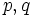# Balanced implies transitive

This article gives the statement and possibly, proof, of an implication relation between two subgroup metaproperties. That is, it states that every subgroup satisfying the first subgroup metaproperty (i.e., Balanced subgroup property (function restriction formalism) (?)) must also satisfy the second subgroup metaproperty (i.e., Transitive subgroup property (?))
View all subgroup metaproperty implications | View all subgroup metaproperty non-implications

## Statement

Suppose$p$ is a balanced subgroup property with respect to the function restriction formalism. In other words, there exists a function property$a$ such that we can write:$p = a \to a$.

Specifically, a subgroup$H$ of a group$G$ satisfies property$p$ in$G$ if and only if every function from$G$ to$G$ satisfying property$a$ restricts to a function from$H$ to$H$ satisfying property$a$.

Then,$p$ is a transitive subgroup property. In other words, if$H \le K \le G$ are subgroups such that$H$ has property$p$ in$K$ and$K$ has property$p$ in$G$, then$H$ has property$p$ in$G$.

## Related facts

### Converse

A converse of sorts is true:

## Definitions used

### Function restriction expression

Further information: function restriction expression

Suppose$a,b$ are properties of functions from a group to itself. Then, the property:$p := a \to b$

is defined as follows. A subgroup$H$ of a group$G$ has property$p$ in$G$ if whenever$f: G \to G$ is a function satisfying property$a$,$f$ restricts to a function from$H$ to$H$ and the restriction of$f$ to$H$ satisfies property$b$.

### Balanced subgroup property

Further information: Balanced subgroup property (function restriction formalism)

A subgroup property$p$ is termed balanced with respect to the function restriction formalism if there exists a function property$a$ such that:$p = a \to a$.

### Composition operator

Further information: composition operator

Suppose$p, q$ are subgroup properties. The composition of these properties, denoted$p * q$, is defined as follows: A subgroup$H$ of a group$G$ is said to have property$p*q$ in$G$ if and only if there exists a subgroup$K$ such that$H \le K \le G$, and such that$H$ has property$p$ in$K$ and$K$ has property$q$ in$G$.

## Examples

Here are some examples of balanced subgroup properties that are also therefore transitive:

Endomorphism$\to$ Endomorphism.

This is balanced. Thus, the property of being fully characteristic is transitive. For full proof, refer: Full invariance is transitive

Automorphism$\to$ Automorphism.

Thus, the property of being a characteristic subgroup is transitive. For full proof, refer: Characteristicity is transitive

Inner automorphism$\to$ Inner automorphism

Thus, the property of being a central factor is transitive. For full proof, refer: Central factor is transitive

## Facts used

1. Composition rule for function restriction:If$a, b, c, d$ are function properties such that$b \le c$, then:$(c \to d) * (a \to b) \le a \to d$

Here,$*$ denotes the composition operator for subgroup properties.

## Proof

Given: A balanced subgroup property$p = a \to a$.

To prove:$p * p \le p$.

Proof: Apply fact (1) with all four variables set to equal$a$. We get:$(a \to a) * (a \to a) \le a \to a$.

This yields the required result:$p * p \le p$.